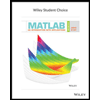# A genetic experiment involving peas yielded one sample of offspring consisting of 455 green peas and 176 yellow peas. Use a 0.05 significance level to test the claim that under the same circumstances, 24 % of offspring peas will be yellow. Identify the null hypothesis, alternative hypothesis, test statistic, P-value, conclusion about the null hypothesis, and final conclusion that addresses the original claim. Use the P-value method and the normal distribution as an approximation to the binomial distribution.

Question

A genetic experiment involving peas yielded one sample of offspring consisting of 455 green peas and 176 yellow peas. Use a 0.05 significance level to test the claim that under the same circumstances, 24 % of offspring peas will be yellow. Identify the null hypothesis, alternative hypothesis, test statistic, P-value, conclusion about the null hypothesis, and final conclusion that addresses the original claim. Use the P-value method and the normal distribution as an approximation to the binomial distribution.

Expert Solution

### Want to see the full answer?

Check out a sample Q&A hereStudents who’ve seen this question also like:MATLAB: An Introduction with Applications
6th Edition
ISBN: 9781119256830
Author: Amos Gilat
Publisher: John Wiley & Sons Inc